Courses

# Test: ILD And Rolling Loads -2

## 10 Questions MCQ Test Topicwise Question Bank for GATE Civil Engineering | Test: ILD And Rolling Loads -2

Description
This mock test of Test: ILD And Rolling Loads -2 for GATE helps you for every GATE entrance exam. This contains 10 Multiple Choice Questions for GATE Test: ILD And Rolling Loads -2 (mcq) to study with solutions a complete question bank. The solved questions answers in this Test: ILD And Rolling Loads -2 quiz give you a good mix of easy questions and tough questions. GATE students definitely take this Test: ILD And Rolling Loads -2 exercise for a better result in the exam. You can find other Test: ILD And Rolling Loads -2 extra questions, long questions & short questions for GATE on EduRev as well by searching above.
QUESTION: 1

Solution:
QUESTION: 2

Solution:
QUESTION: 3

### The absolute maximum Bending Moment in a simply supported beam of span 20 m due to a moving UDL of 4 t/m spanning over 5 m is

Solution:

Absolute maximum bending moment will occur at the centre when the load is spread equally on either side of the centre.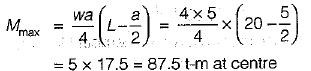QUESTION: 4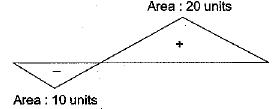​Influence line diagram for a truss member is shown in the above figure. Positive values indicate tension. Dead load of the truss is 20 kN/m and the live load is 10 kN/m. Live load is longer than the span. Maximum tensile force in the member is:

Solution:

Tensile force due to D.L.
= (20 - 10) x 20 = 200 kN
Maximum Tensile force due to L.L.
=  20 x 10 = 200 kN
Thus maximum tensile force
= 200 + 200 = 400 kN

QUESTION: 5

The influence line for horizontal thrust of a two- hinged parabolic arch of span i’ and rise 'h' will be shown in

Solution:

Figure in (a) is the I.L.D. of horizontal, thrust for three hinged arch

QUESTION: 6

A Warren truss is supported as shown in the given figure. Which one of the following diagrams represents the influence line for the force in member U3U4?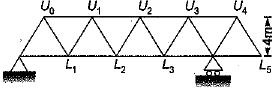Solution:

Introduce a cut through U3U4, U3L4and L3L4. The member forces of L3L4 and U3L4 pass through support at L4 . So w hen load is at L0, L1 L2, L3 and L4. the total moment of all the force except force in U3U4 on the right part of cut section is zero. So from moment equilibrium the force in U3U4 will remain zero when load is between. L0 and L4.
When load is at L5, the force in member U3U4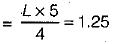QUESTION: 7

A simply-supported beam with overhangs is shown in the figure. The influence line diagram for shear in respect of a section just to the right of the support 'A wiil be as shown in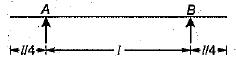Solution:
QUESTION: 8

A simply supported beam is shown in the figure-I. The influence line for the beam is drawn in figure-II, Which one of the following statements is correctly associated with the figure-II?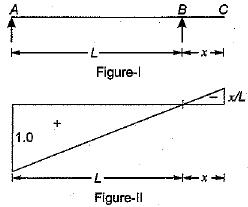Solution:

Since the unit value of I.L.D. is 1,0 at A. So this is for reaction at support A

QUESTION: 9

Consider the following statements:
1. An influence line for a function (example: moment shear force, reaction, deflection) in a structure is a curve which shows its variation at a particular section of the structure for various positions of a moving unit load.
2. The influence line for bending moment/shear force must not be confused with bending moment diagram and shear force diagram for the structure.
3. The bending moment diagram and shear force diagram shows the moment/shear values at all sections of the structure. The influence line diagram for BM/SF is always drawn for a moving unit point load and for a particular section only

Of these statements:

Solution:
QUESTION: 10

Which one of the following equations represents influence line of fixed end moment at B of the fixed beam AB of length l with origin at A?

Solution:

For the influence line of fixed end moment at B, release the moment at B and give unit rotation, the deformed shape will represent the influence line.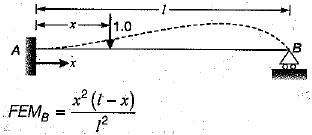due to unit load at x distance from A.